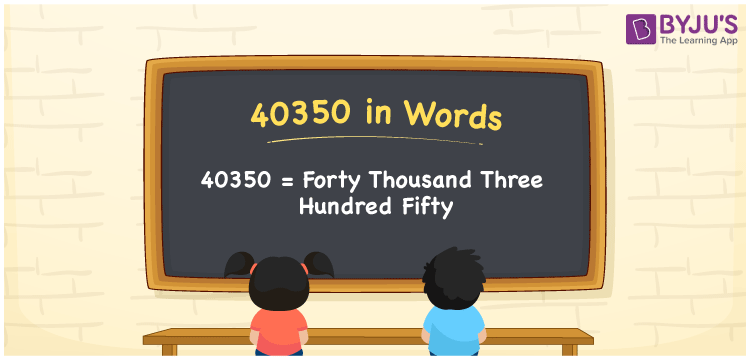# 40350 in Words

40350 in words is written as Forty thousand three hundred fifty. In both the International System of Numerals and the Indian System of Numerals, 40350 is written as Forty thousand three hundred fifty. The number 40350 is a Cardinal Number as it represents some quantity. For example, that laptop costs 40350 rupees.

 40350 in Words Forty thousand three hundred fifty Forty thousand three hundred fifty in Number 40350

## 40350 in English Words

40350 in English words is read as “Forty thousand three hundred fifty”.## How to Write 40350 in Words?

To write 40350 in words, we shall use the place value chart. In the place value chart, put 4 in the ten thousands, 0 in the thousands, 3 in the hundreds, 5 in the tens and 0 in the ones, respectively. Let us make a place value chart to write the number 40350 in words.

 Ten Thousands Thousands Hundreds Tens Ones 4 0 3 5 0

Thus, we can write the expanded form as

4 × Ten Thousand + 0 × Thousand + 3 × Hundred + 5 × Ten + 0 × One

= 4 × 10000 + 0 × 1000 + 3 × 100 + 5 × 10 + 0 × 1

= 40000 + 0 + 300 + 50 + 0

= 40350

= Forty thousand three hundred fifty.

40350 is a natural number, the successor of 40349 and the predecessor of 40351.

40350 in words – Forty thousand three hundred fifty

• Is 40350 an odd number? – No
• Is 40350 an even number? – Yes
• Is 40350 a perfect square number? – No
• Is 40350 a perfect cube number? – No
• Is 40350 a prime number? – No
• Is 40350 a composite number? – Yes

## Frequently Asked Questions on 40350 in Words

Q1

### How to write 40350 in words?

40350 in words is written as Forty thousand three hundred fifty.
Q2

### How to write 40350 in words in the International and Indian System of Numerals?

In both, the system of numerals, 40350 in words, is written as Forty thousand three hundred fifty.
Q3

### How to write 40350 in a place value chart?

In the place value chart, write 4 in the ten thousands, 0 in the thousands, 3 in the hundreds, 5 in the tens and 0 in the ones, respectively.